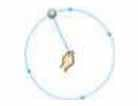# 6th Std Science Term 1 Solution | Lesson.2 Force and Motion

## Lesson.2 Force and Motion## Lesson.2 Force and Motion

### I. Choose the correct answer:

1. Unit of speed is

1. m
2. s
3. kg
4. m/s

Ans : m/s

2. Oscillatory motion among the following is

1. Rotation of the earth about its axis
2. Revolution of the moon about the earth
3. To and fro movement of a vibrating string
4. All of these.

Ans : To and fro movement of a vibrating string

3. The correct relation among the following is

1. Speed = distance × time
2. Speed = distance / time.
3. Speed = time / distance
4. Speed = 1 / (distance × time)

Ans : Speed = distance / time.

4. Gita rides with her father’s bike to her uncle’s house which is 40 km away from her home. She takes 40 minutes to reach there.

Statement 1 : She travels with a speed of 1 km / minute.

Statement 2 : She travels with a speed of 1 km/hour

1. Statement 1 alone is correct.
2. Statement 2 alone is correct.
3. Both statement 1 and 2 are correct.
4. Neither statement 1 nor statement 2 is correct.

Ans : Statement 1 alone is correct.

### II . Find whether the following statements are true or false. – if false give the correct answer

1. To and fro motion is called oscillatory motion.

Ans : True

2. Vibratory motion and rotator motion are periodic motion.

Ans : False

Cu. An : Vibratory motion and oscillatory motion are periodic motions.

3. Vehicles moving with varying speeds are said to be in uniform motion.

Ans : False

Cu. An : Vehicles moving with varying speeds are said to be in non-uniform motion.

4. Robots will replace human in future

Ans : False

Cu. An : Robots will not replace human in future.

### III. Fill up the blanks.

1. A bike moving on a straight road is an example of ……….. motion

Ans : linear

2. Gravitational force is a …………….. force

Ans : rotatory

3.  Motion of a potter’s wheel is an example of …………….. motion

Ans : rotatory

4. When an object covers equal distances in equal interval of time, it is said to be in …………….. motion

Ans : uniform

### IV. Analogy.

1. kicking a ball : contact force :: falling of leaf : …………………………… ?

Ans : non contact force

2. Distance : metre :: speed : ………………………….. ?

Ans : metre/ second

3. circulatory motion :: a spinning top :: oscillatory motion : …………………………… ?

Ans : swinging of a pendulum

### V. Match the followingCircular motionOscillatory motionlinear motionrotatory motionlinear and rotator motion Ans : 1 – C, 2 – D, 3 – B, 4 – A, 5 – E

### VI.Give one word for the following statements .

1. The force which acts on an object without Physical contact with it.

Non contact force

2. A change in the position of an object with time.

Motion

3. The motion which repeats itself after a fixed interval of time.

Oscillatory motion

4. The motion of an object travels equal distances in equal intervals of time.

Uniform motion

5. A machine capable of carrying out a complex series of actions automatically.

Robots.

### VII. Answer the following in a sentence or two

1. Define force.

Forces are push or pull by an animate or inanimate agency.

2. Name different types of motion based on the path.

• Linear motion
• Curvilinear motion
• Circular motion
• Rotatory motion
• Oscillatory motion
• Zigzag (irregular) motion

3. If you are sitting in a moving car, will you be at rest or motion with respect to your friend sitting next to you?

I am in rest with respect to my friend, sitting inside the car.

4. Rotation of the earth is a periodic motion. Justify.

Motion repeated in equal intervals of time is called as periodic motion. The earth rotates on its axis once in 24 hours. The duration of time is fixed as 24 hours. Therefore the rotation of earth is a periodic motion.

5. Differentiate between rotational and curvilinear motion.

 Rotational Motion Curvilinear Motion. 1. A body moves along a circular path. A body moves along a curved path. 2. Without changing its position, about its own (fixed) axis. Changes its position with motion. Eg. Rotation of a spinning top. Eg. Throwing paper airplanes or paper darts.

### VIII. Calculate

1. A vehicle covers a distance of 400km in 5 hour. Calculate its average speed

 Distance covered by the vehicle = 400 km Time taken = 5 hours Average Speed = distance covered / time taken = 400 𝑘𝑚 / 5 ℎ𝑜𝑢𝑟 = 80 km/hr

1. What is motion? Classify different types of motion with examples.

Motion: Change of position of any object with respect to time is known as motion.

### 1. Based on path:

#### i) Linear motion

• eg. Parade of the soldiers.

#### ii) Curvilinear motion

• eg. Paper flight moving.

#### iii) Circular motion

• eg. Swirling stone tied to the rope

#### iv) Rotatory motion

• eg. Rotating top

#### v) Oscillatory motion

• eg. Clock pendulum

#### vi) Zigzag (irregular) motion

• eg. Motion of a bee

### 2. Based on duration:

#### i) Periodic motion

• eg. Motion of a bob of simple pendulum

#### ii) Non periodic motion

• eg. swaying of the branches of a tree.

### 3. Based on speed:

#### i) Uniform motion

eg. Hour hand of a clock

#### ii) Non-uniform motion

• eg. Motion of a train, as it leaves a station.